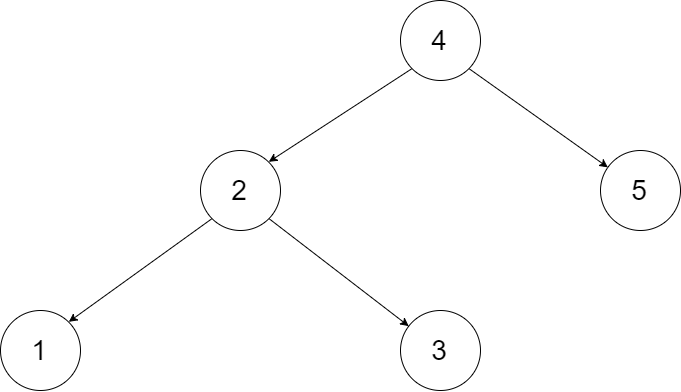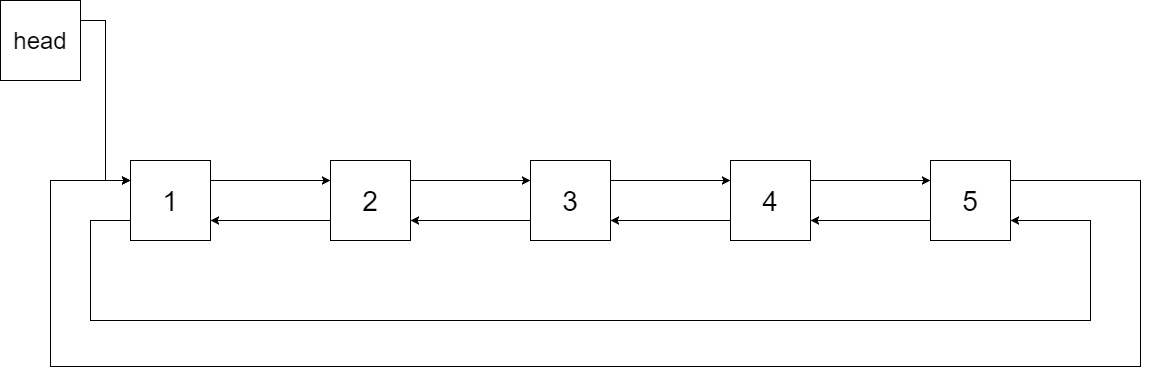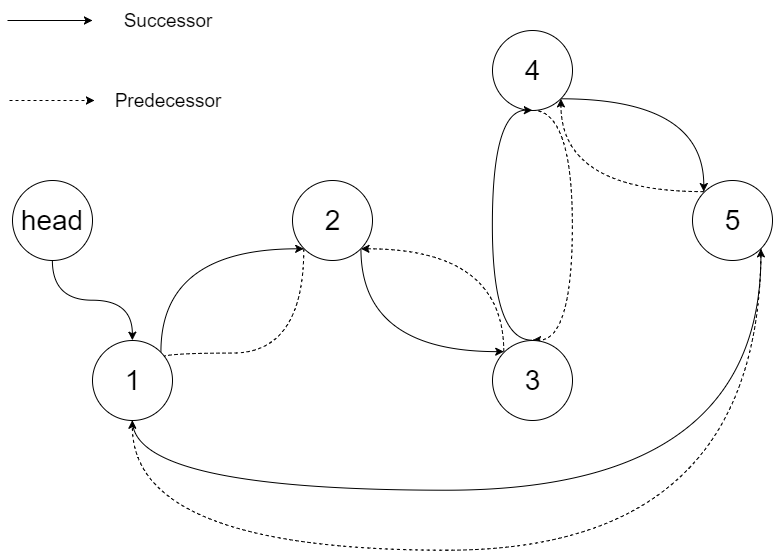# LeetCode: 426. 将二叉搜索树转化为排序的双向链表¶

## 1、题目描述¶## 2、解题思路¶

• 使用基于栈的中序遍历
• 首先创建一个head作为前驱节点
• 设置一个前驱结点pre，每次都是将前面的pre和当前节点进行左右链接
• 最后处理head与最后一个节点之间的链接即可
"""
# Definition for a Node.
class Node:
def __init__(self, val, left=None, right=None):
self.val = val
self.left = left
self.right = right
"""
from collections import deque

class Solution:
def treeToDoublyList(self, root: 'Node') -> 'Node':
if not root:
return root

stack = deque()
stack.append(root)
while stack:
while stack[-1].left:
stack.append(stack[-1].left)
while stack:
current = stack.pop()
current.left = pre
pre.right = current
pre = current
# 操作
if current.right:
stack.append(current.right)
break